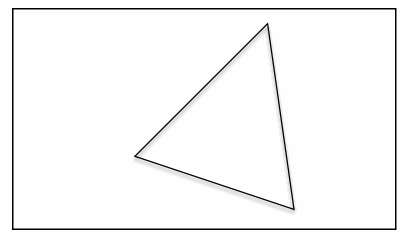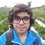# Geometry Challenge: Drawing Polygons in a RectangleWe are given a rectangle with dimensions $l \times w$, where $l$ and $w$ are positive real numbers and $l \geq w$. In this note, we will try to find the area of the largest regular polygons that can be drawn completely inside the rectangle.

A shape is said to be drawn completely inside the rectangle if no point of the shape is outside the rectangle.

$\color{gold} {\text{Level 1}}$
What is the area of the largest regular quadrilateral that can be drawn completely inside the rectangle?

$\color{#EC7300} {\text{Level 2}}$
What is the area of the largest regular triangle that can be drawn completely inside the rectangle?

$\color{#D61F06} {\text{Level 3}}$
What is the area of the largest regular hexagon that can be drawn completely inside the rectangle?

$\color{#69047E}{\text{Level 4}}$
Prove (or disprove): The largest possible regular $n$-gon that can be drawn completely in the rectangle is drawn. If $n$ is even, then two opposite sides of the $n$-gon will lie on longer sides $l$ of the rectangle.

$\color{#3D99F6}{ \text{Level 5}}$
Prove (or disprove) : There exists a general formula to calculate the area of the largest regular $n$-gon that can be drawn completely inside the rectangle in terms of $l, w,$ and $n$.

If such a formula exists, find it.Note by Pranshu Gaba
6 years ago

This discussion board is a place to discuss our Daily Challenges and the math and science related to those challenges. Explanations are more than just a solution — they should explain the steps and thinking strategies that you used to obtain the solution. Comments should further the discussion of math and science.

When posting on Brilliant:

• Use the emojis to react to an explanation, whether you're congratulating a job well done , or just really confused .
• Ask specific questions about the challenge or the steps in somebody's explanation. Well-posed questions can add a lot to the discussion, but posting "I don't understand!" doesn't help anyone.
• Try to contribute something new to the discussion, whether it is an extension, generalization or other idea related to the challenge.

MarkdownAppears as
*italics* or _italics_ italics
**bold** or __bold__ bold
- bulleted- list
• bulleted
• list
1. numbered2. list
1. numbered
2. list
Note: you must add a full line of space before and after lists for them to show up correctly
paragraph 1paragraph 2

paragraph 1

paragraph 2

[example link](https://brilliant.org)example link
> This is a quote
This is a quote
    # I indented these lines
# 4 spaces, and now they show
# up as a code block.

print "hello world"
# I indented these lines
# 4 spaces, and now they show
# up as a code block.

print "hello world"
MathAppears as
Remember to wrap math in $$ ... $$ or $ ... $ to ensure proper formatting.
2 \times 3 $2 \times 3$
2^{34} $2^{34}$
a_{i-1} $a_{i-1}$
\frac{2}{3} $\frac{2}{3}$
\sqrt{2} $\sqrt{2}$
\sum_{i=1}^3 $\sum_{i=1}^3$
\sin \theta $\sin \theta$
\boxed{123} $\boxed{123}$

Sort by:

Level 5: I think formula is $\frac{n\omega ^2}{4} tan(\frac{\pi}{n})$ for even .I m not sure coz i calculated it orally so i could have missed something conceptually too.

For odd: $\frac{n \omega ^2 sin(\frac{2 \pi}{n})}{2(1+ cos(\frac{\pi}{n}))^2}$

- 6 years ago

Hmm, something is not right. Your formulae are independent of $l$. However, the final result does depend on $l$. You may try the level 2 problem first to see the dependence on $l$.

Could you elaborate on how you obtained these formulae? Thanks.

- 6 years ago

Hey can u tell me how even one will depend on $l$.

- 6 years agoLet diagnol be D. Hence $Dcosa=\omega$ Area of square =$1/2 D^2$ =$\frac{w^2}{2cos^2a}$.Now cos is a decreasing function and max value of $a$ is 45 degress coz after that figure will come out of rectangle and at 45 degree opp sides will be rectangle's longer sides.

This can be extended to general .Let there be n sides so angle subtented at centre will be $\frac{2\pi}{n}$ Let the Longest diagnol be D and it also makes an angle a with vertical .Hence $Dcosa=w$.Now area of one triangle

=$\frac{(D/2)^2 Sin(2\pi/n)}{2}$

So n triangles area= $\frac{nd^2 Sin(\frac{2\pi}{n})}{8}$= $\frac{nw^2 Sin(\frac{2\pi}{n})}{8cos^2(a)}$

similarily max value of total area will occur at $a=\pi /n$ because cos is a decreasing function and after $\pi/n$ figure will come out of rectangle and also 2 opp sides will be on longer sides of rectangle. Simplifying it we get the formula i stated below for even.Note i have taken two points on longer opp sides to generalise and then shown for max that two sides of polygon have to be on two longer sides of rectangle.

can u please tell why it will depend on $l$

- 6 years ago

Your reasoning is correct for a square. In a square, $a$ should be $45^{\circ}$. Area of square will be $\frac{w^2}{ 2 \cos^{2}a} = w^2$, and it will not depend on $l$. However, this reasoning does not extend for other $n$.

Consider a hexagon. Following the same reasoning, the longest diagonal will make an angle $\pi / 6$ with the vertical. The length of one side of the hexagon will be $\frac{w}{\sqrt{3}}$. The width of the hexagon will be $\frac{2w}{\sqrt{3}}$. If $l > \frac{2w}{\sqrt{3}}$, then area does not depend on $l$. However, if $l$ is less than $\frac{2w}{\sqrt{3}}$, then some of the hexagon will go out of the rectangle, then the area does depend on $l$.

- 6 years ago

OOps!correct I think i should sleep .I m sickk

- 6 years ago

Nice efforts though!

- 6 years ago